# Ewald sum

The Ewald sum technique  was originally developed by Paul Ewald to evaluate the Madelung constant . It is now widely used in order to simulate systems with long range interactions (typically, electrostatic interactions). Its aim is the computation of the interaction of a system with periodic boundary conditions with all its replicas. This is accomplished by the introduction of fictitious "charge clouds" that shield the charges. The interaction is then divided into a shielded part, which may be evaluated by the usual means, and a part that cancels the introduction of the clouds, which is evaluated in Fourier space.

## Derivation

In a periodic system one wishes to evaluate the internal energy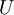$U$ (Eq. 1.1 ):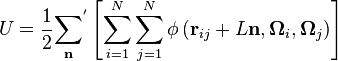$U = \frac{1}{2} {\sum_{\mathbf n}}^{'} \left[ \sum_{i=1}^N \sum_{j=1}^N \phi \left({\mathbf r}_{ij} + L{\mathbf n}, {\mathbf \Omega_i}, {\mathbf \Omega_j} \right) \right]$

where one sums over all the simple cubic lattice points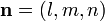${\mathbf n} = (l,m,n)$. The prime on the first summation indicates that if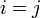$i=j$ then the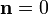${\mathbf n} = 0$ term is omitted.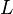$L$ is the length of the side of the cubic simulation box,$N$ is the number of particles, and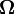${\mathbf \Omega}$ represent the Euler angles.

This internal energy is partitioned into four contributions: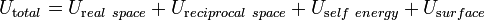$U_{\mathrm total} = U_{\mathrm real~space} + U_{\mathrm reciprocal~space} + U_{\mathrm self~energy} + U_{\mathrm surface}$

#### Real-space term

The real space contribution to the electrostatic energy is given by  (Eq. 7a and 7b ):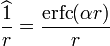$\widehat{\frac{1}{r}} = \frac{\mathrm {erfc}(\alpha r)}{r}$

where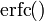${\mathrm {erfc}}()$ is the complementary error function, and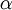$\alpha$ is the Ewald screening parameter. Also,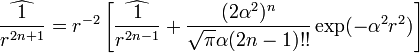$\widehat{ \frac{1}{r^{2n+1}} } = r^{-2} \left[ \widehat{ \frac{1}{r^{2n-1}} } + \frac{(2\alpha^2)^n}{ \sqrt{\pi} \alpha (2n-1)!! } \exp(-\alpha^2r^2) \right]$

## Particle mesh

#### Smooth particle mesh (SPME)

SPME. Optimisation  .